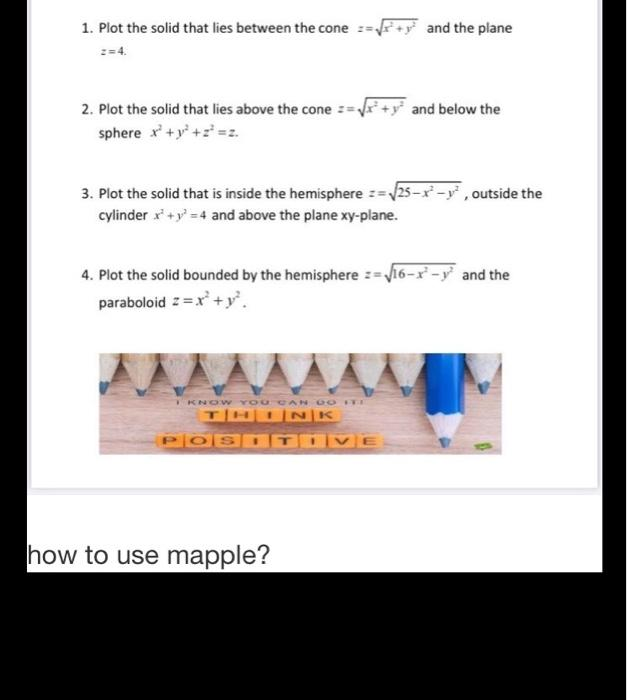### Create an Account

Home / Questions / 1. Plot the solid that lies between the cone *+y? and the plane and below the 2. Plot the ...

# 1. Plot the solid that lies between the cone *+y? and the plane and below the 2. Plot the solid that lies above the cone sphere x + y + z =2. 3. Plot the solid that is inside the hemisphere := 25-8-y,

1. Plot the solid that lies between the cone *+y? and the plane and below the 2. Plot the solid that lies above the cone sphere x + y + z =2. 3. Plot the solid that is inside the hemisphere := 25-8-y, outside the cylinder x' + y =4 and above the plane xy-plane. 4. Plot the solid bounded by the hemisphere := V16-r-y and the paraboloid 2 = xº+y?. I KNOW YOU CAN DO THINK POSTOVE how to use mapple?May 07 2021 View more View LessSubscribe To Get Solution